# Data ellipses and confidence ellipses

This just clarifies the distinction between a data ellipse and a confidence ellipse, i.e. an ellipse describing the joint confidence intervals on two parameters of a model

Chris Evans https://www.psyctc.org/R_blog/ (PSYCTC.org)https://www.psyctc.org/psyctc/
01-15-2022

# History

• Created 15.i.22
• Updated 16.i.22 adding ggExtra::ggMarginal() plots

Follows the typically generous and helpful post from John Fox on the R-help list:

``````Dear Paul,

On 2022-01-14 1:17 p.m., Paul Bernal wrote:
> Dear John and R community friends,
>
> To be a little bit more specific, what I need to accomplish is the
> creation of a confidence interval ellipse over a scatterplot at
> different percentiles. The confidence interval ellipses should be drawn
> over the scatterplot.

I'm not sure what you mean. Confidence ellipses are for regression
coefficients and so are on the scale of the coefficients; data
(concentration) ellipses are for and on the scale of the explanatory
variables. As it turns out, for a linear model, the former is the
rescaled 90 degree rotation of the latter.

Because the scatterplot of the (two) variables has the variables on the
axes, a data ellipse but not a confidence ellipse makes sense (i.e., is
in the proper units). Data ellipses are drawn by car::dataEllipse() and
(as explained by Martin Maechler) cluster::ellipsoidPoints(); confidence
ellipses are drawn by car::confidenceEllipse() and the various methods
of ellipse::ellipse().

I hope this helps,
John``````

That made me realise that I was only “sort of” sure I understood that and reminded me that I have so far never used ellipses either as a way to describe 2D data or to map the confidence intervals of two parameters from a model. I decided to get to grips with this, starting by creating some correlated data.

Show code
``````set.seed(12345) # get replicability
valN <- 300 # sample size (doh!)
x <- rnorm(valN) # Gaussian distribution
y <- x + rnorm(valN, sd = .3) # create correlated y variable
bind_cols(x = x, y = y) -> tibDat # build into a tibble
``````

Here’s the head of that dataset.

Show code
``````### show the data
tibDat
``````
``````# A tibble: 300 × 2
x       y
<dbl>   <dbl>
1  0.586  0.742
2  0.709  0.712
3 -0.109 -0.241
4 -0.453 -0.0937
5  0.606  0.571
6 -1.82  -1.81
7  0.630  0.989
8 -0.276 -0.173
9 -0.284 -0.383
10 -0.919 -0.418
# … with 290 more rows``````

And here is a simple ggplot scattergram of that using transparency to handle overprinting.

Show code
``````### simple scattergram
ggplot(data = tibDat,
aes(x = x, y = y)) +
geom_point(alpha = .4) +
geom_smooth(method = "lm") +
xlim(c(-3, 3)) +
ylim(c(-3, 3)) +
coord_fixed(1) -> p
p
``````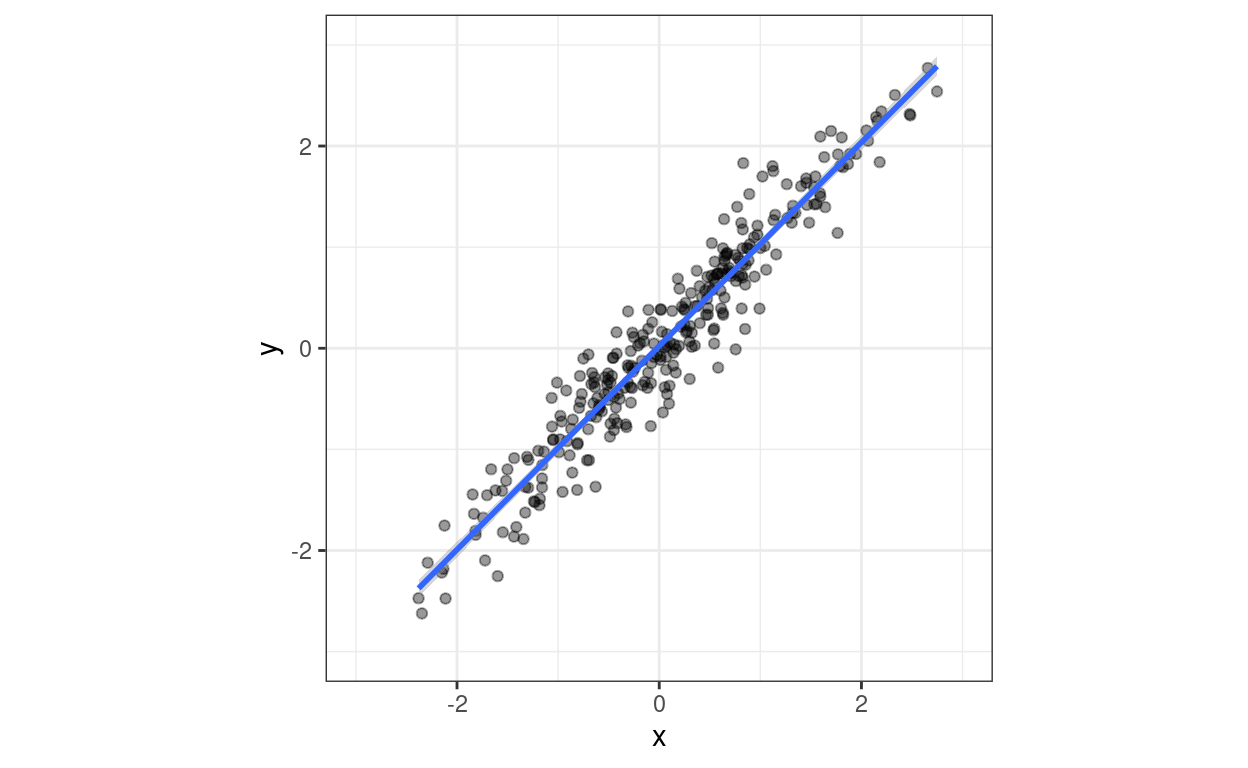### ggExtra::ggMarginal() adds marginal histograms

This is just so I remember where to find this and for the fun of it: `ggExtra::ggMarginal()` can add marginal histograms, density plots, boxplots, violin plots or “densigrams”, a combination of a histogram and a density plot, to the sides of a scattergram. I like this!

#### “Densigram”

Show code
``````ggExtra::ggMarginal(p, type = "densigram")
``````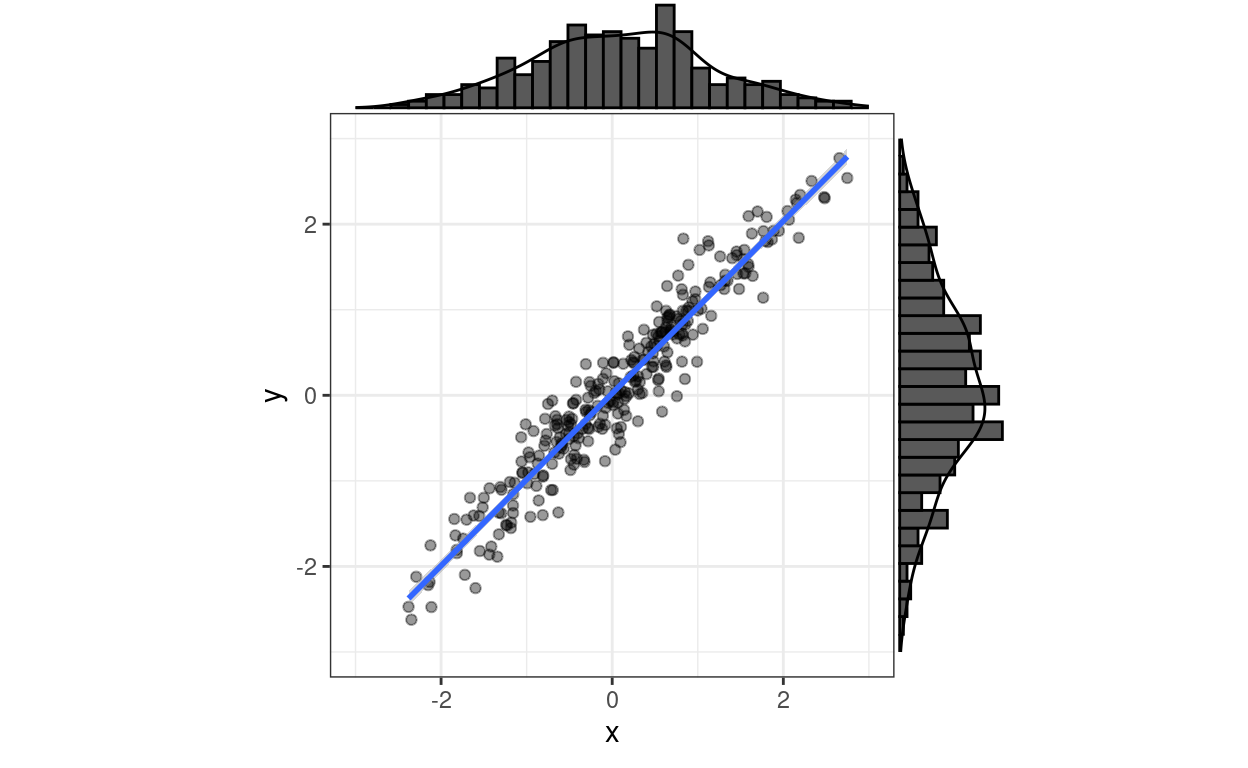#### Boxplot

Show code
``````ggExtra::ggMarginal(p, type = "boxplot")
``````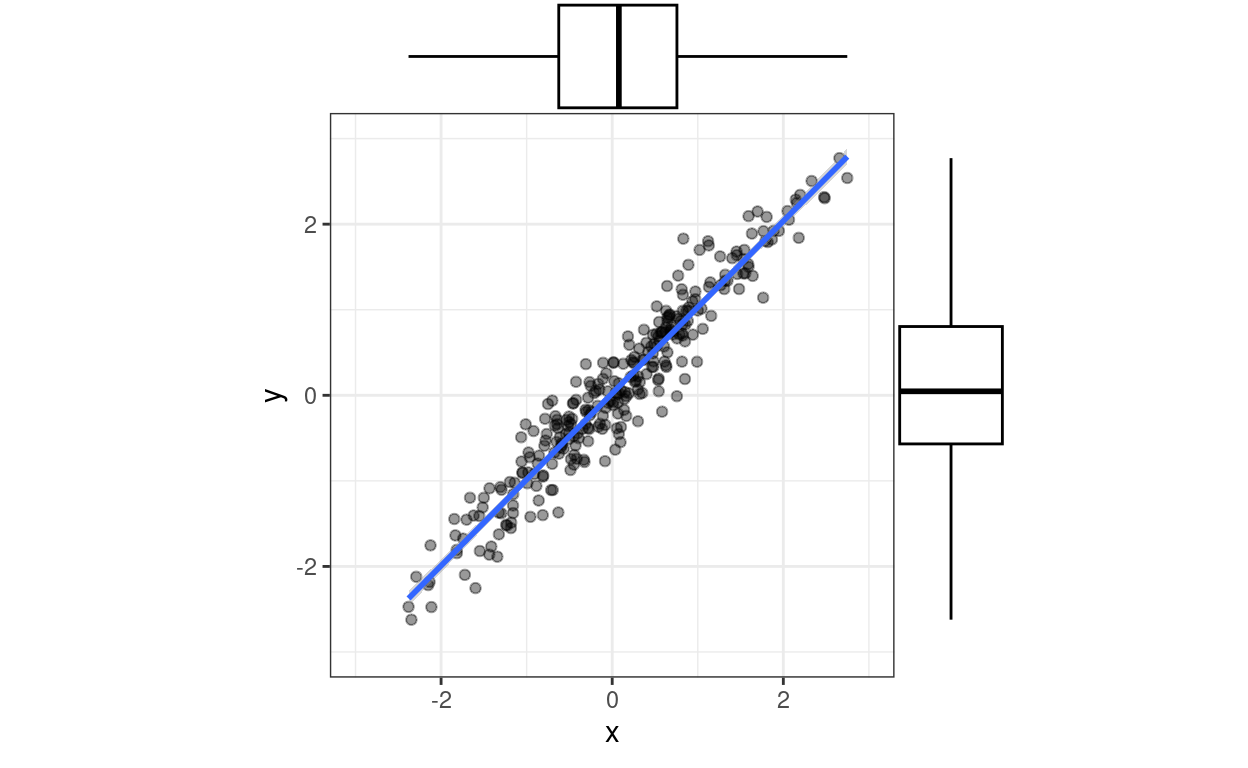#### Violin plot

Show code
``````ggExtra::ggMarginal(p, type = "violin")
``````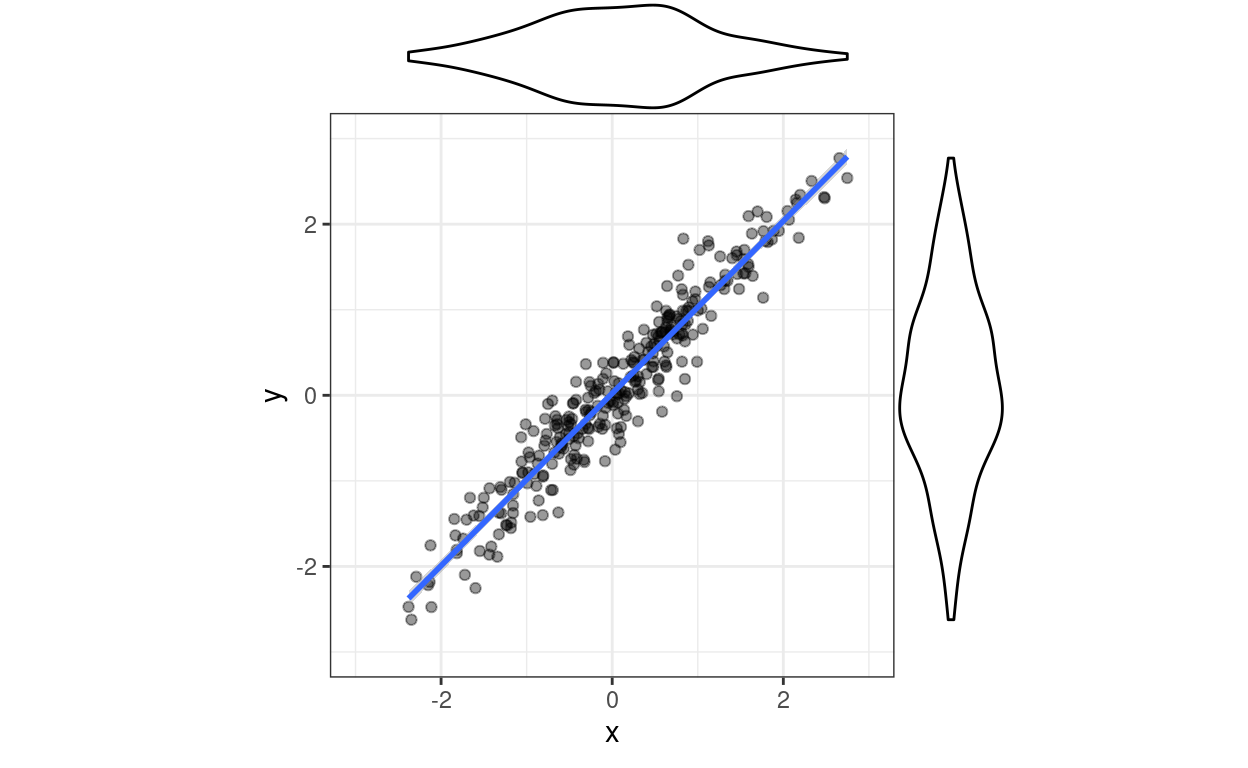OK, back to the main issue.

## Data ellipses

A 95% data ellipse is an ellipse expected to contain 95% of the joint population distributions of x and y based on the observed data and the assumption of bivariate Gaussian distributions. The area contained can be what you like really (within the logical restrictions of it being a positive proportion/percentage and lower than 100%!) Here are data ellipses for that dataset created with `car::dataEllipse()`. I’ve used its default confidence intervals of 50% and 95%.

Show code
``````car::dataEllipse(tibDat\$x, tibDat\$y) -> retDat # collect up data for the lines
``````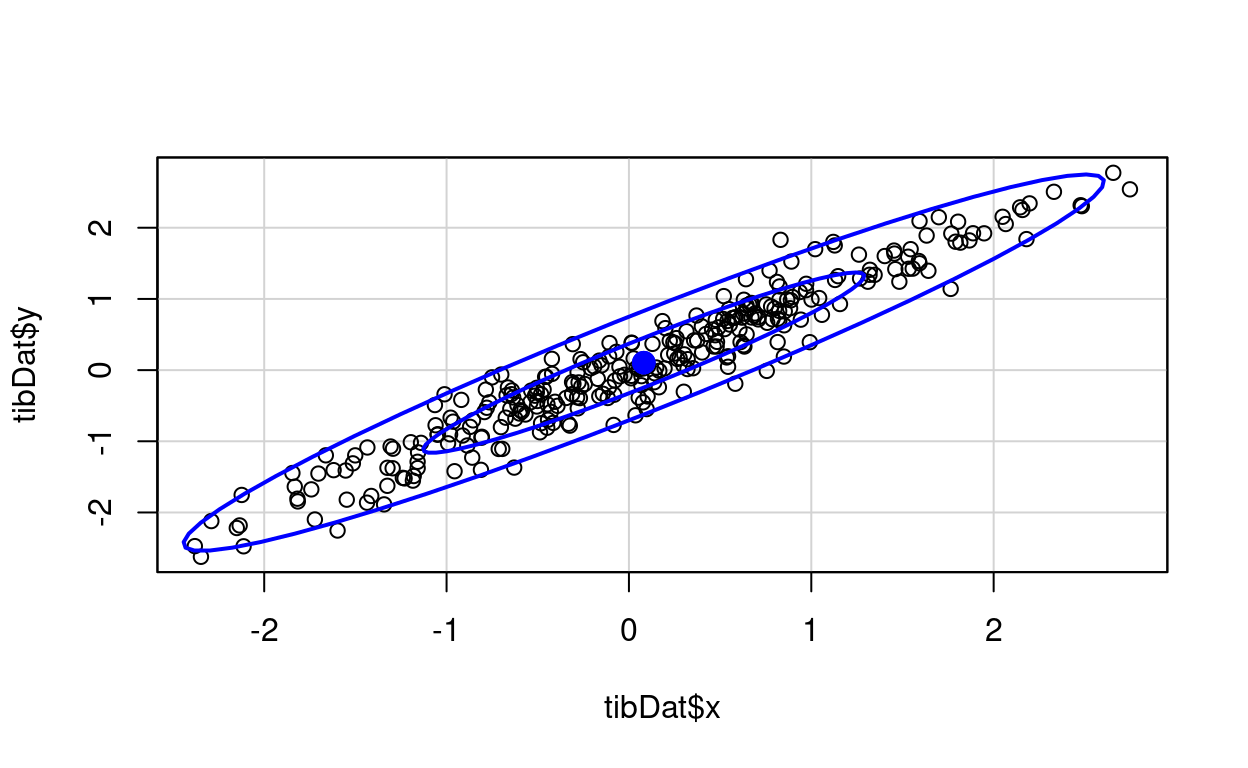Show code
``````# str(retDat)
### retDat is a list containing a mapping for the ellipses
### 50% ellipse points
retDat\$`0.5` %>%
as_tibble() -> tib50
### 95% ellipse points
retDat\$`0.95` %>%
as_tibble() -> tib95
``````

Here’s the same but using `cluster::ellipsoidPoints()`. A bit more work than `car::dataEllipse()`.

Show code
``````tibDat %>%
as.data.frame() %>%
as.matrix() -> matDat

matCovLS <- cov(matDat)
vecMeans <- colMeans(matDat)
vecMeans <- colMeans(matDat)
### get 95% CI ellipse
d2.95 <- qchisq(0.95, df = 2)
cluster::ellipsoidPoints(matCovLS, d2.95, loc = vecMeans) -> matEllipseHull95
### and now 50%
d2.50 <- qchisq(0.5, df = 2)
cluster::ellipsoidPoints(matCovLS, d2.50, loc = vecMeans) -> matEllipseHull50

plot(matDat, asp = 1, xlim = c(-3, 3))
lines(matEllipseHull95, col="blue")
lines(matEllipseHull50, col="blue")
``````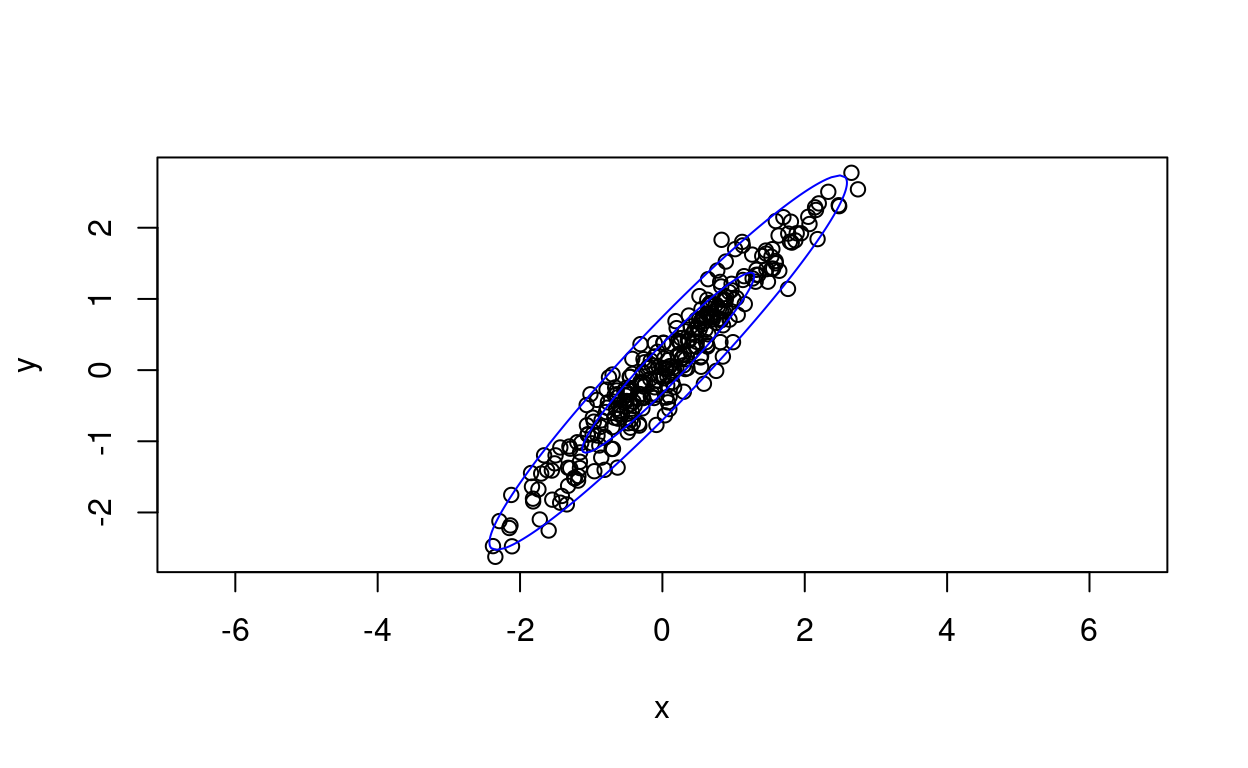That really is the same as the other, well, minus the 50% interval but it looks different because of the changed scales.

### “Robust” data ellipse

Just to extend things, the help for `cluster::ellipsoidPoints()` shows that you can use it with a “robust covariance” estimate rather than the least squares `lm()` or `cov()` one. Turns out that this uses `cov.rob()` from the MASS package which essentially does some censoring off of perceived or potential outliers to get an covariance matrix that would be less sensitive to outliers. Here we go.

Show code
``````Cxy <- MASS::cov.rob(cbind(x,y))
cluster::ellipsoidPoints(Cxy\$cov, d2 = d2.95, loc=Cxy\$center) -> matEllipseHullRob

plot(matDat, asp = 1, xlim = c(-3, 3))
lines(matEllipseHull95, col="blue")
lines(matEllipseHullRob, col="green")
``````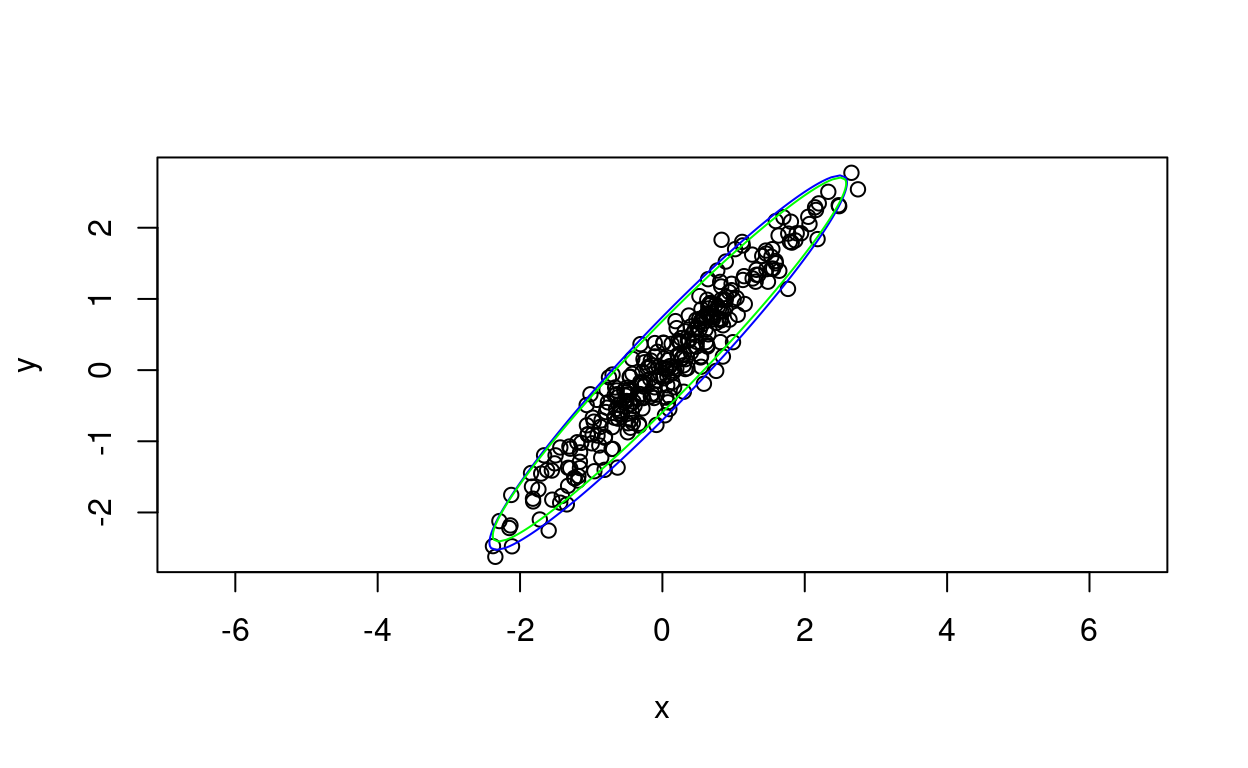That has the 95% ellipse from the robust covariance matrix in green and the simple least squares ellipse in blue. As you would expect the difference is negligible as these are bivariate Gaussian data so there are few real outliers.

These plots are reminding me that all that learning curve to understand ggplot was worth it! However, the corollary is that I have forgotten most of what I ever knew about improving base R graphic output. Fortunately, I can take the output from `car::dataEllipse()` and feed it into ggplot where I use `geom_path()` to plot it.

Show code
``````ggplot(data = tibDat,
aes(x = x, y = y)) +
geom_point(alpha = .4) +
geom_smooth(method = "lm") +
xlim(c(-3, 3)) +
ylim(c(-3, 3)) +
coord_fixed(1) +
geom_path(data = tib50,
aes(x = x, y = y), colour = "red") +
geom_path(data = tib95,
aes(x = x, y = y), colour = "orange")
``````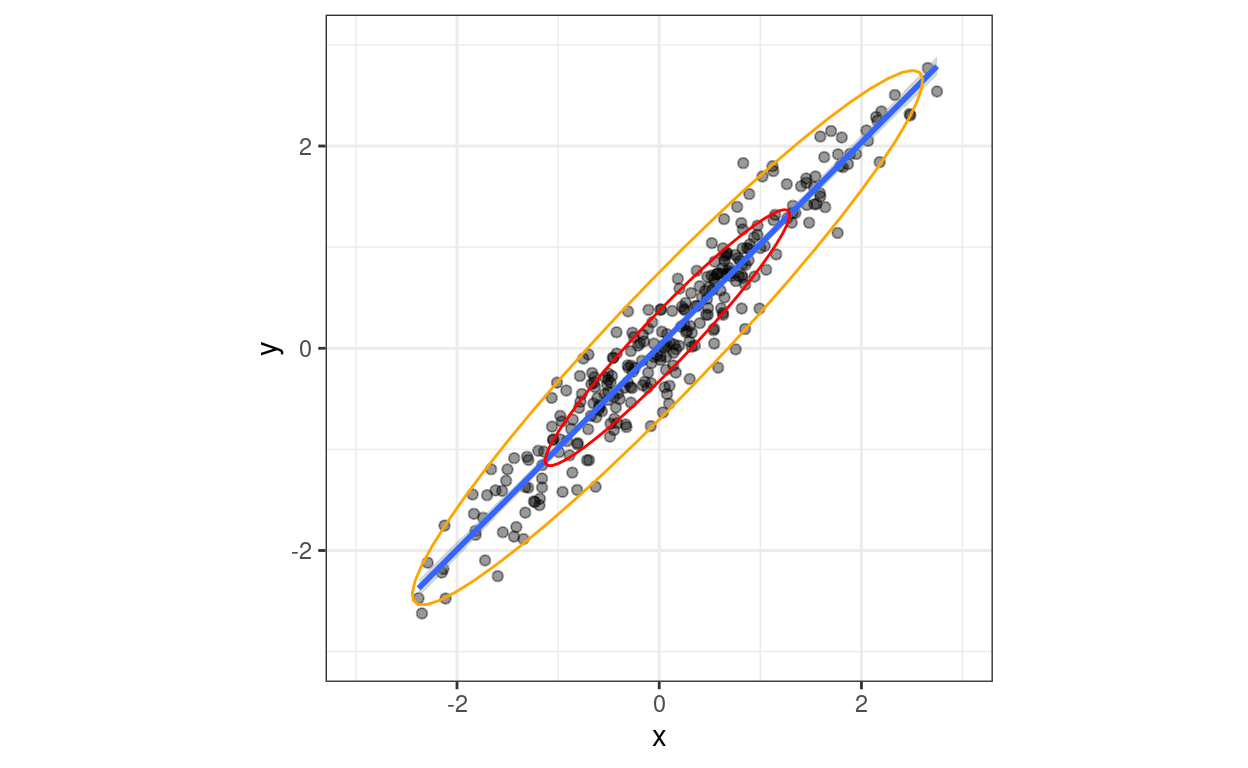So that’s same again, now just feeding the points created by `car::dataEllipse()` for each CI into tibbles and those into ggplot and overlaying them on the scattergram.

### Ellipsoid hulls (or ellipsoidhulls)

This was an interesting extension of my learning. An ellipsoid hull is different from a data ellipse: it’s the ellipse that contains all the observed points (with some on the boundary of the ellipse). Here using `cluster::ellipsoidhull()` and base graphics.

Show code
``````tibDat %>%
as.data.frame() %>%
as.matrix() -> matDat

cluster::ellipsoidhull(matDat) -> ellipseHull

plot(matDat, asp = 1, xlim = c(-3, 3))
lines(predict(ellipseHull), col="blue")
``````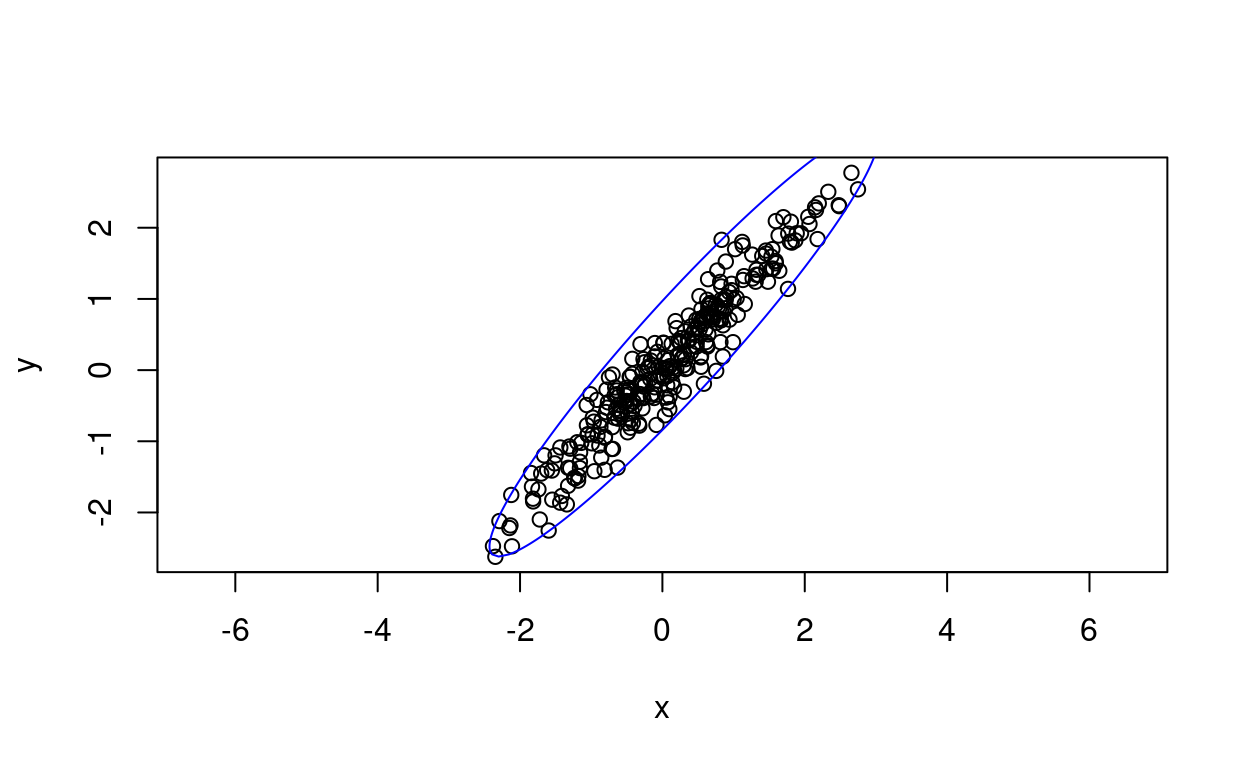And the same spitting the data into ggplot.

Show code
``````predict(ellipseHull) %>%
as_tibble(.name_repair = "universal") %>%
rename(x = `...1`) -> tibEllipseHullPath

ggplot(data = tibDat,
aes(x = x, y = y)) +
geom_point(alpha = .4) +
xlim(c(-4, 4)) +
ylim(c(-4, 4)) +
coord_fixed(1) +
geom_path(data = tibEllipseHullPath,
aes(x = x, y = y), colour = "blue")
``````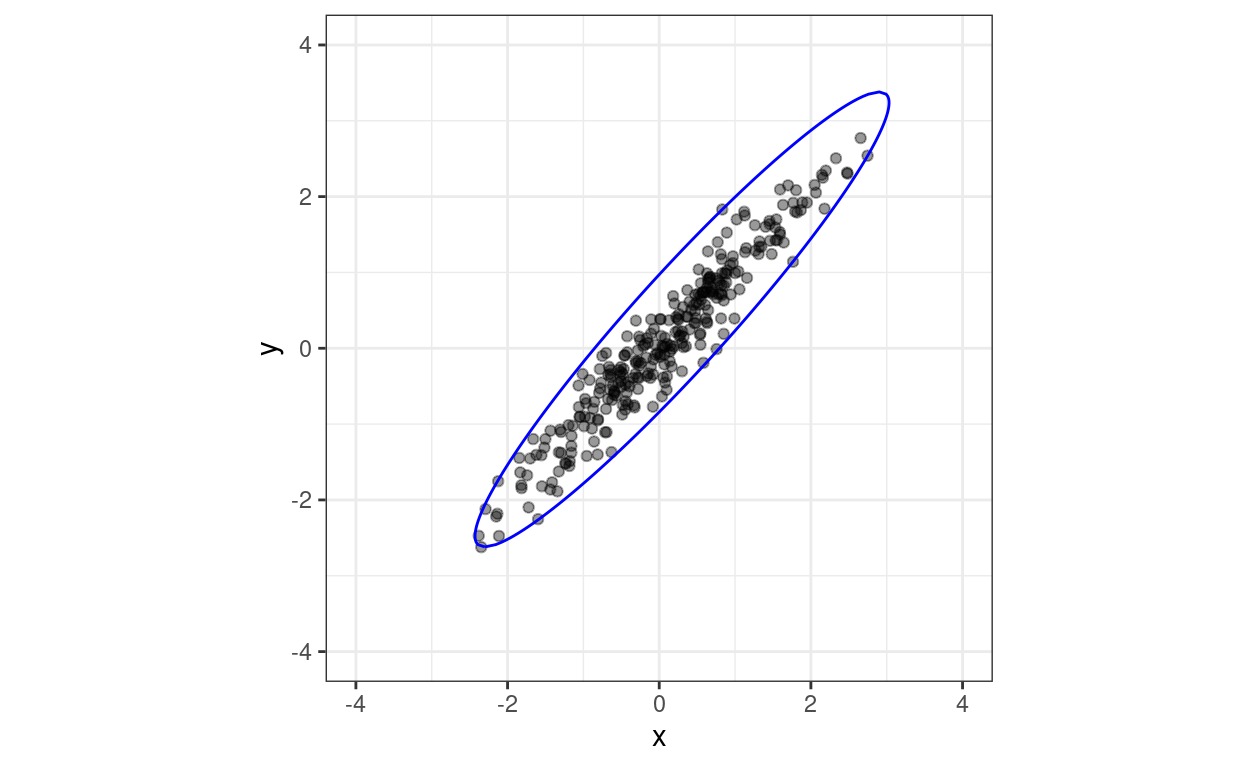## Confidence ellipses

So what are confidence ellipses? These are not about estimation the distribution of the population data but confidence ellipses for model parameters estimated from the data. Here the model is linear regression of y on x and assuming Gaussian distributions and here are the model parameters estimated using `lm()`.

Show code
``````lm(y ~ x, data = tibDat)
``````
``````
Call:
lm(formula = y ~ x, data = tibDat)

Coefficients:
(Intercept)            x
0.02265      1.00701  ``````

The two parameters are the intercept and the slope and the confidence ellipse shows the area containing the desired joint CIs. The default interval is 95% and here it is constructed using `car::confidenceEllipse()`. The point in the middle marks the point estimates of intercept and slope and the ellipse the CI around that.

Show code
``````car::confidenceEllipse(lm(y ~ x, data = tibDat))
``````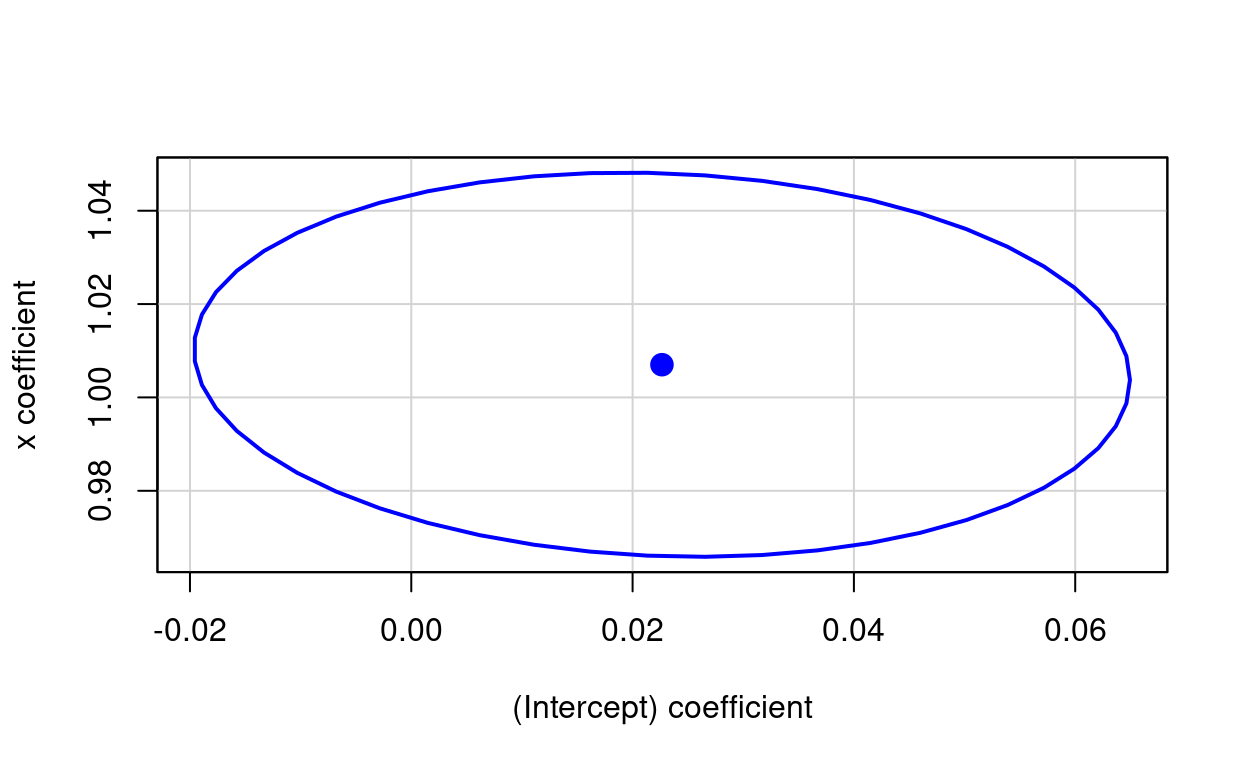Here is the same ellipse created using `ellipse::ellipse()`.

Show code
``````ellipse::ellipse(lm(y ~ x, data = tibDat)) -> matEllipseEllipse
plot(ellipse::ellipse(lm(y ~ x, data = tibDat)), type = "l")
``````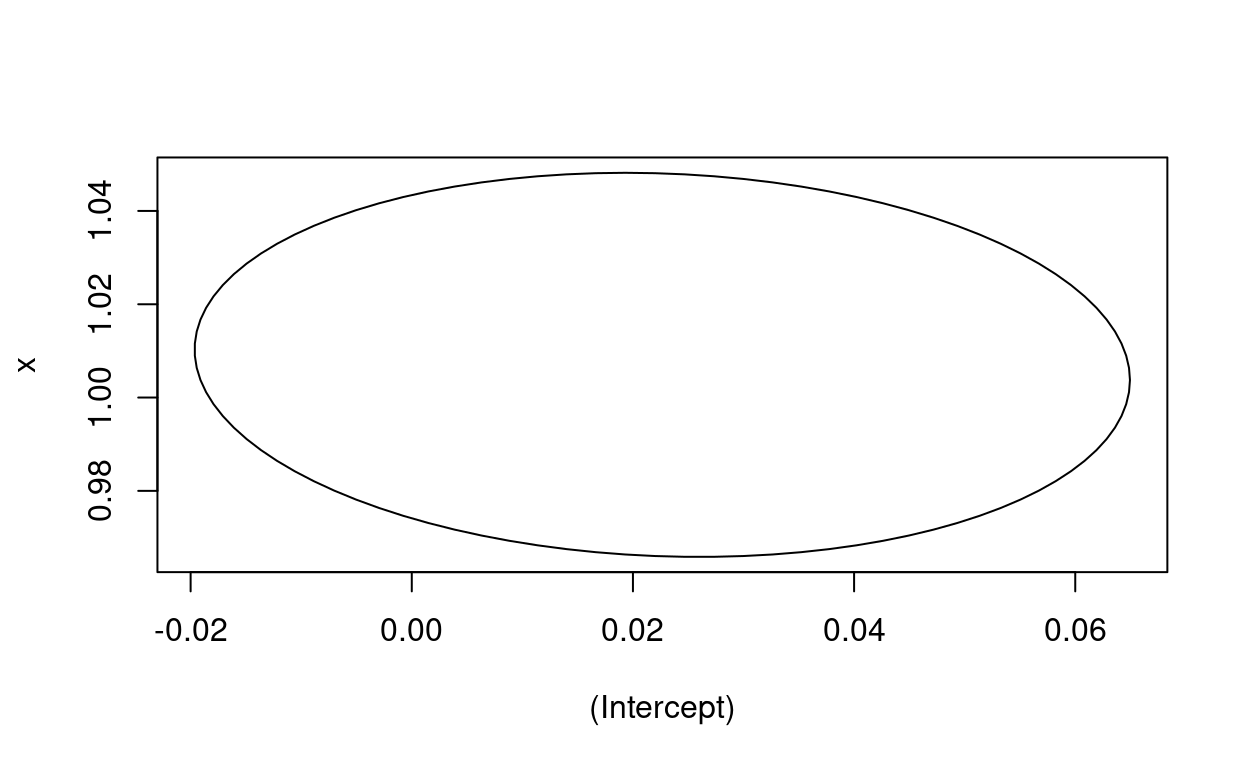Just for completeness, it’s easy to get the ellipse path using `ellipse::ellipse()` and spit that into ggplot.

Show code
``````ellipse::ellipse(lm(y ~ x, data = tibDat)) -> matEllipseEllipse

### rather clumsy creation of tibble of parameters for ggplot
lm(y ~ x, data = tibDat)\$coefficients -> vecLM
bind_cols(Intercept = vecLM, Slope = vecLM) -> tibParms

### slightly nicer creation of tibble of the points on the ellipse
matEllipseEllipse %>%
as_tibble() %>%
rename(Intercept = `(Intercept)`,
Slope = x) -> tmpTib

### plot those
ggplot(data = tmpTib,
aes(x = Intercept, y = Slope)) +
geom_path() +
geom_point(data = tibParms,
colour = "blue",
size = 3)
``````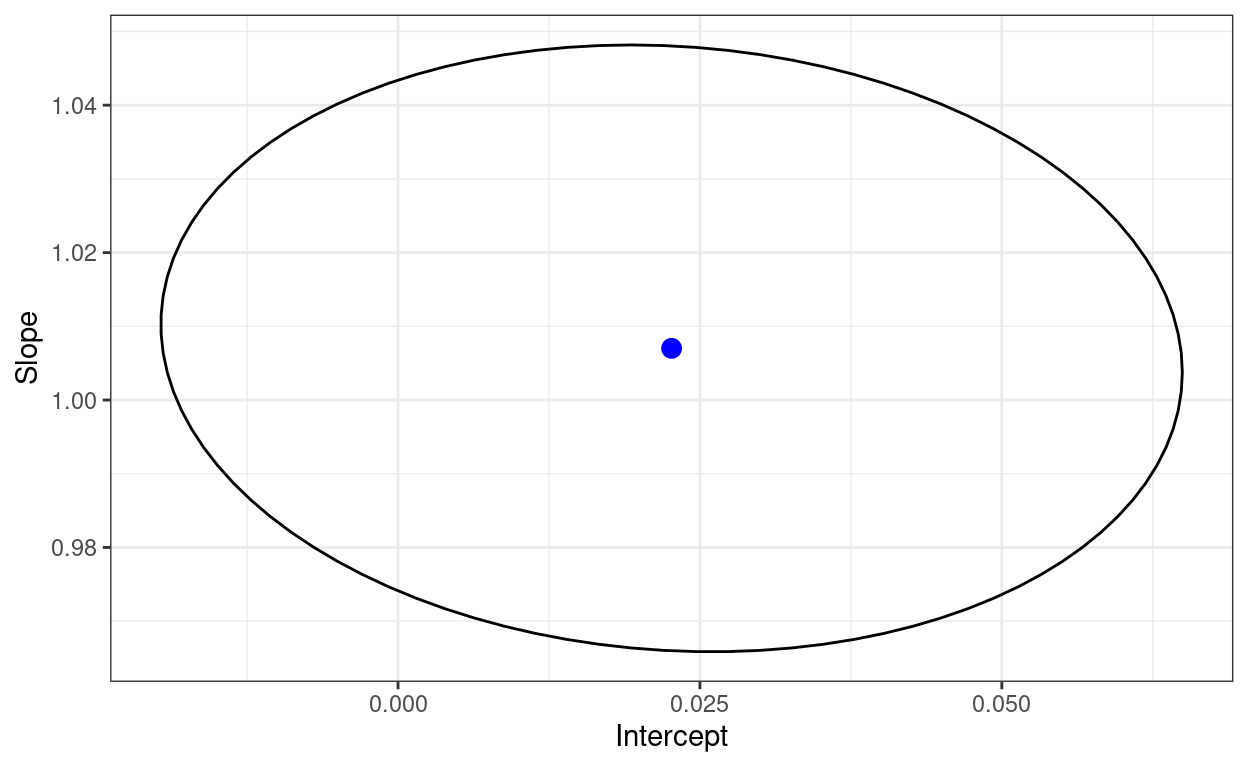That shows a joint distribution suggesting that the two estimated parameters are pretty much uncorrelated. I think that doesn’t have to be the case. Let’s try the very non-Gaussian joint distribution we get if we square both x and y. Here’s the scattergram and 50% and 95% data ellipses for that.

Show code
``````tibDat %>%
mutate(xSqrd = x^2,
ySqrd = y^2) -> tibDat

car::dataEllipse(tibDat\$xSqrd, tibDat\$ySqrd) -> retDat2 # collect up data for the lines
``````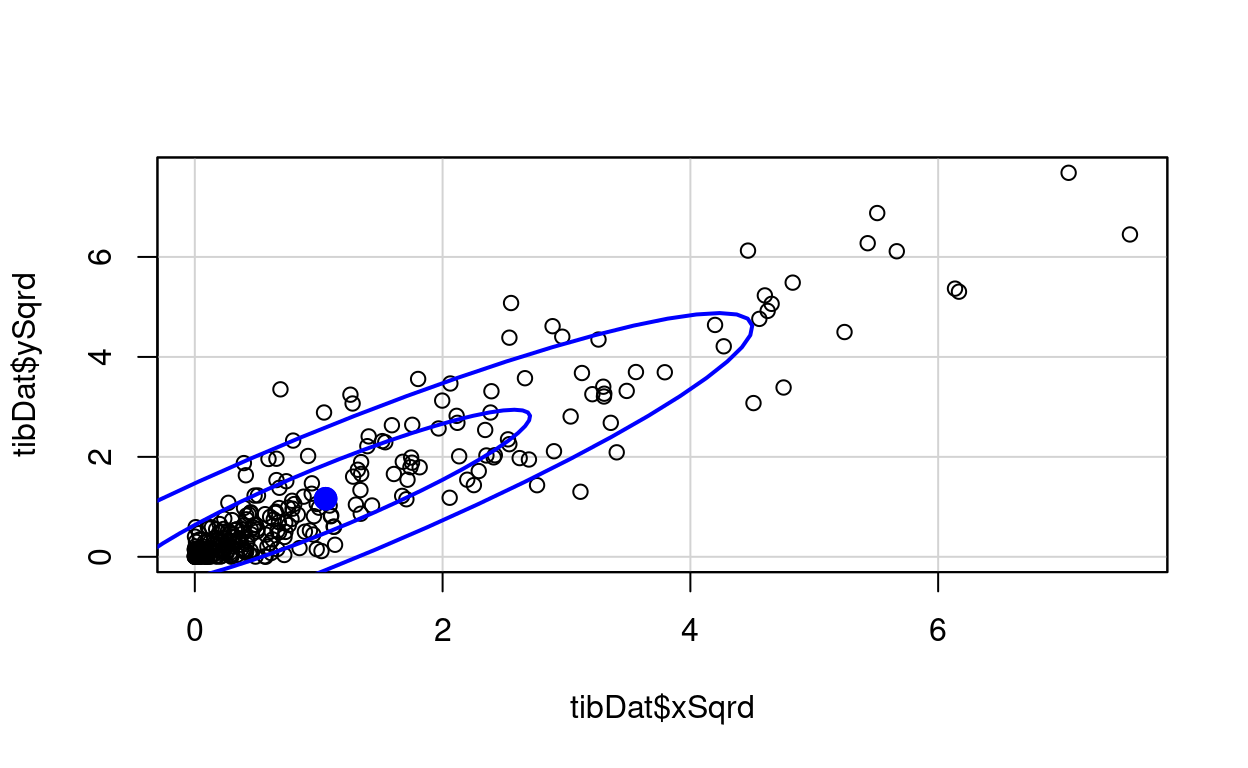Show code
``````# str(retDat)
retDat2\$`0.5` %>%
as_tibble() -> tibSqrd50

retDat2\$`0.95` %>%
as_tibble() -> tibSqrd95

ggplot(data = tibDat,
aes(x = xSqrd, y = ySqrd)) +
geom_point(alpha = .4) +
geom_smooth(method = "lm") +
xlim(c(0, 9)) +
ylim(c(0, 9)) +
coord_fixed(1) +
geom_path(data = tib50,
aes(x = x, y = y), colour = "red") +
geom_path(data = tib95,
aes(x = x, y = y), colour = "orange")
``````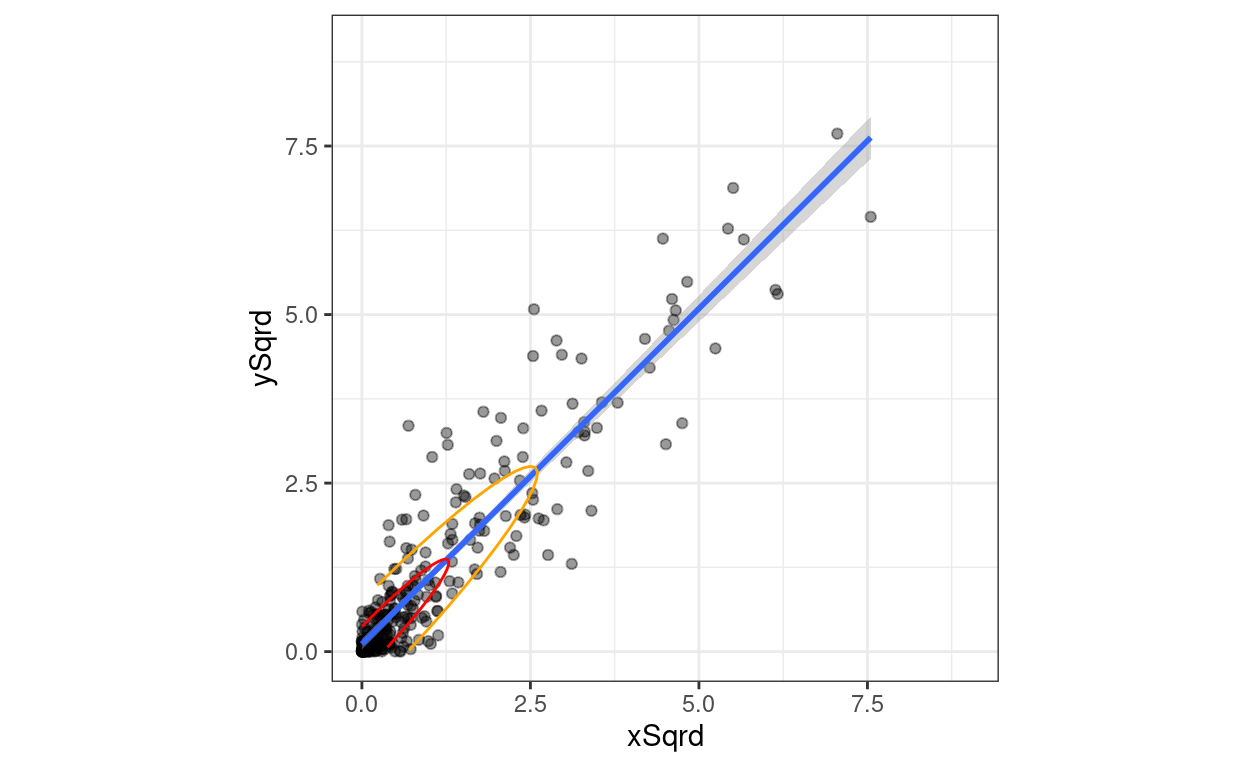And here is the confidence ellipse from `car::confidenceEllipse()`.

Show code
``````car::confidenceEllipse(lm(ySqrd ~ xSqrd, data = tibDat))
``````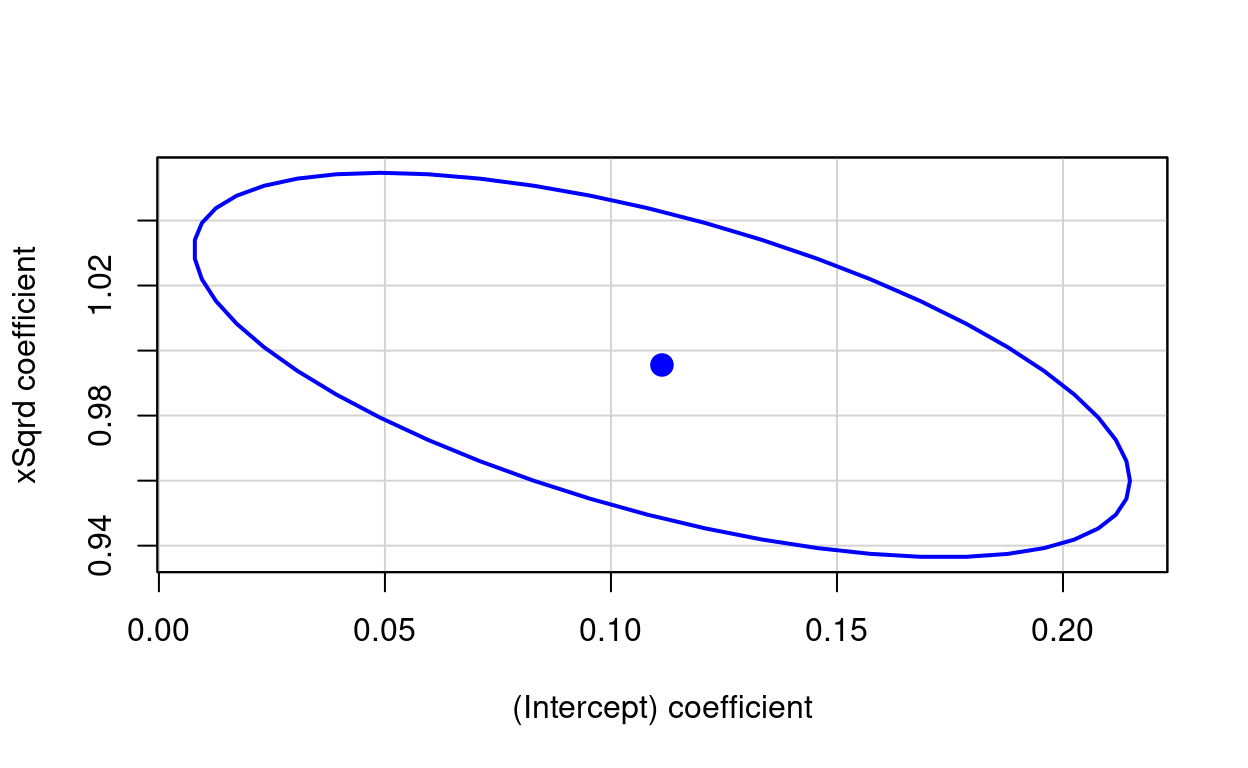Same by ggplot.

Show code
``````ellipse::ellipse(lm(ySqrd ~ xSqrd, data = tibDat)) -> matEllipseEllipse2

### rather clumsy creation of tibble of parameters for ggplot
lm(ySqrd ~ xSqrd, data = tibDat)\$coefficients -> vecLM2
bind_cols(Intercept = vecLM2, Slope = vecLM2) -> tibParms2

### slightly nicer creation of tibble of the points on the ellipse
matEllipseEllipse2 %>%
as_tibble() %>%
rename(Intercept = `(Intercept)`,
Slope = xSqrd) -> tmpTib2

### plot those
ggplot(data = tmpTib2,
aes(x = Intercept, y = Slope)) +
geom_path() +
geom_point(data = tibParms2,
colour = "blue",
size = 3)
``````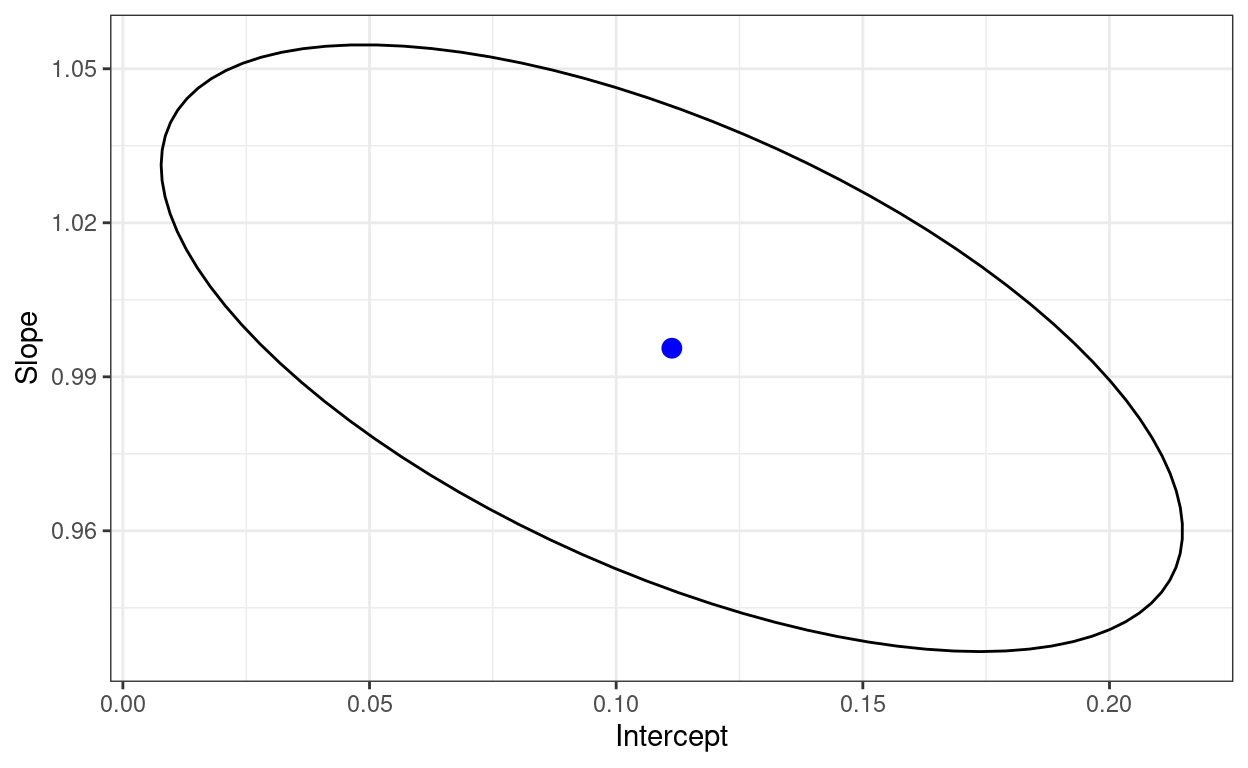OK. I think that’s enough on this!

### Citation

For attribution, please cite this work as

`Evans (2022, Jan. 15). Chris (Evans) R SAFAQ: Data ellipses and confidence ellipses. Retrieved from https://www.psyctc.org/R_blog/posts/2022-01-15-data-ellipses-and-confidence-ellipses/`

BibTeX citation

```@misc{evans2022data,
author = {Evans, Chris},
title = {Chris (Evans) R SAFAQ: Data ellipses and confidence ellipses},
url = {https://www.psyctc.org/R_blog/posts/2022-01-15-data-ellipses-and-confidence-ellipses/},
year = {2022}
}```